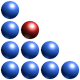# Complexity-based Classification of Dynamical System

The fundamental equation of the QCT shows how complexity itself is a variable with its own dynamics. As the interplay between structure (by structure we intend the map representing the flow of information within the system, i.e. the Complexity Map) and entropy cannot be expressed in the form of a function, this equation cannot be solved in closed form. However, setting the derivative of complexity to zero allows us to distinuish between the two types of above mentioned systems, i.e. isoentropic (entropy is constant) and isomorphic (the structure of the underlying complexity map remains unchanged). In the most general case, a system can find itself functioning as an isoentropic or an isomorphic system or in a situation in which both structure and entropy drive the evolution of complexity.